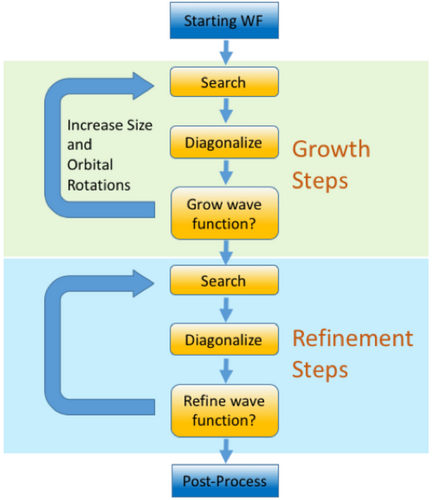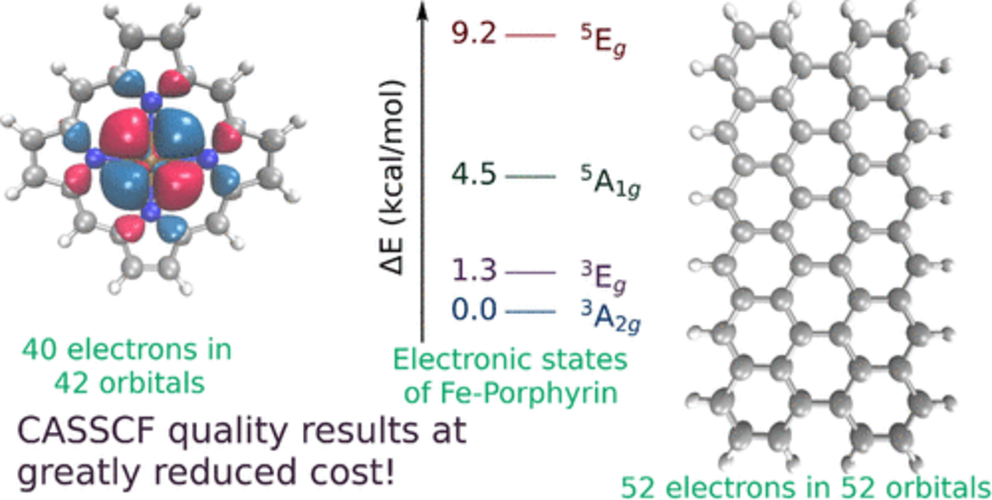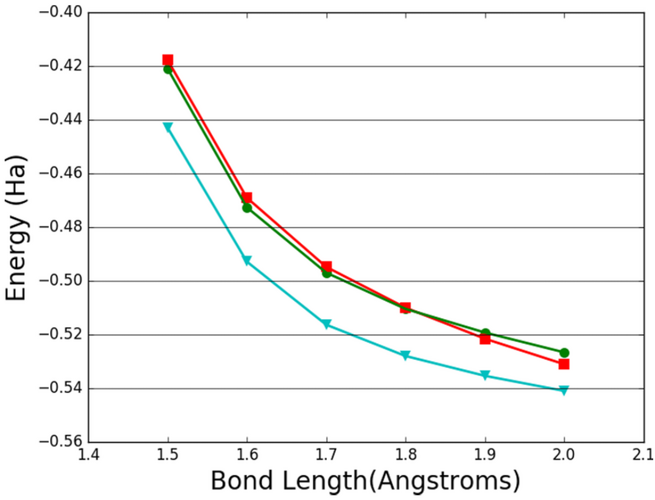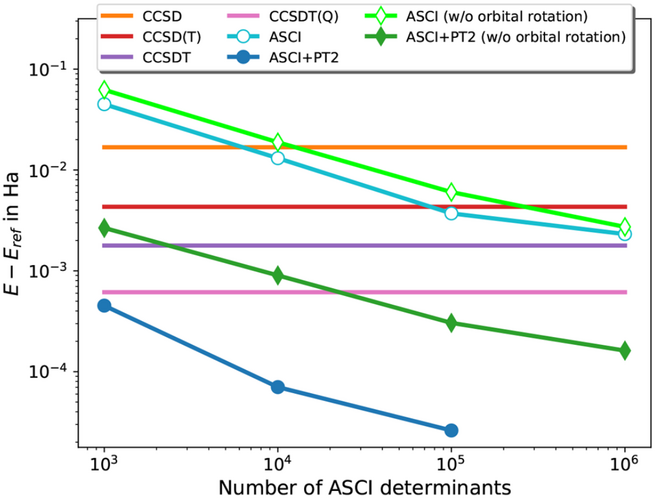# Adaptive Sampling Configuration Interaction (ASCI) Method• ASCI is a compact approximation to the unattainable full CI ansatz. In ASCI, a trial CI wave function is iteratively improved by the inclusion of new determinants for which the estimated CI coefficients are significant.

• The ASCI energy can be further improved through second-order Epstein-Nesbet perturbation theory (PT2).

• The ASCI scheme can be applied in CASSCF calculations (ASCI-SCF), affording much larger active spaces than conventional CASSCF calculations and extending CASSCF calculations to medium-sized molecules.Cr\(_2\) binding curves. Green, ASCI+PT2 energies; red, DRMG energies for (24e,30o); blue, ASCI energies without the frozen core (48e,42o)Convergence rate of ASCI and ASCI+PT2 energies for the CN radical in the cc-pVDZ basis, with increasing size of ASCI wave functions